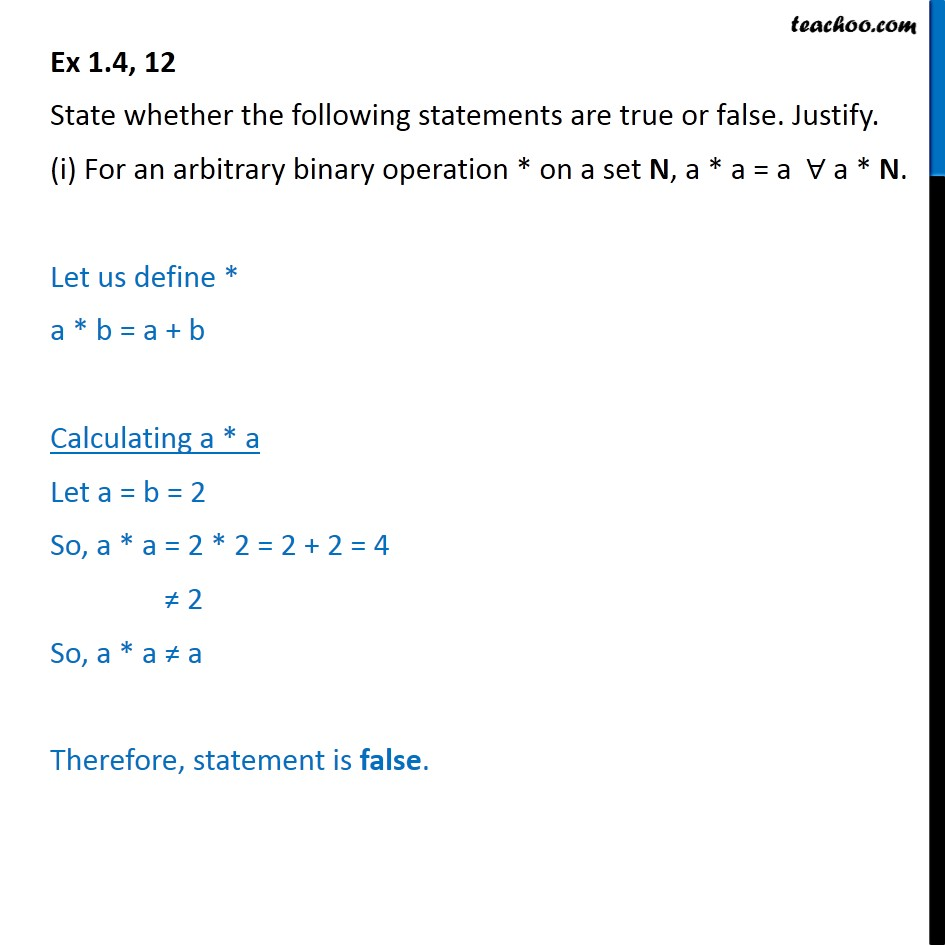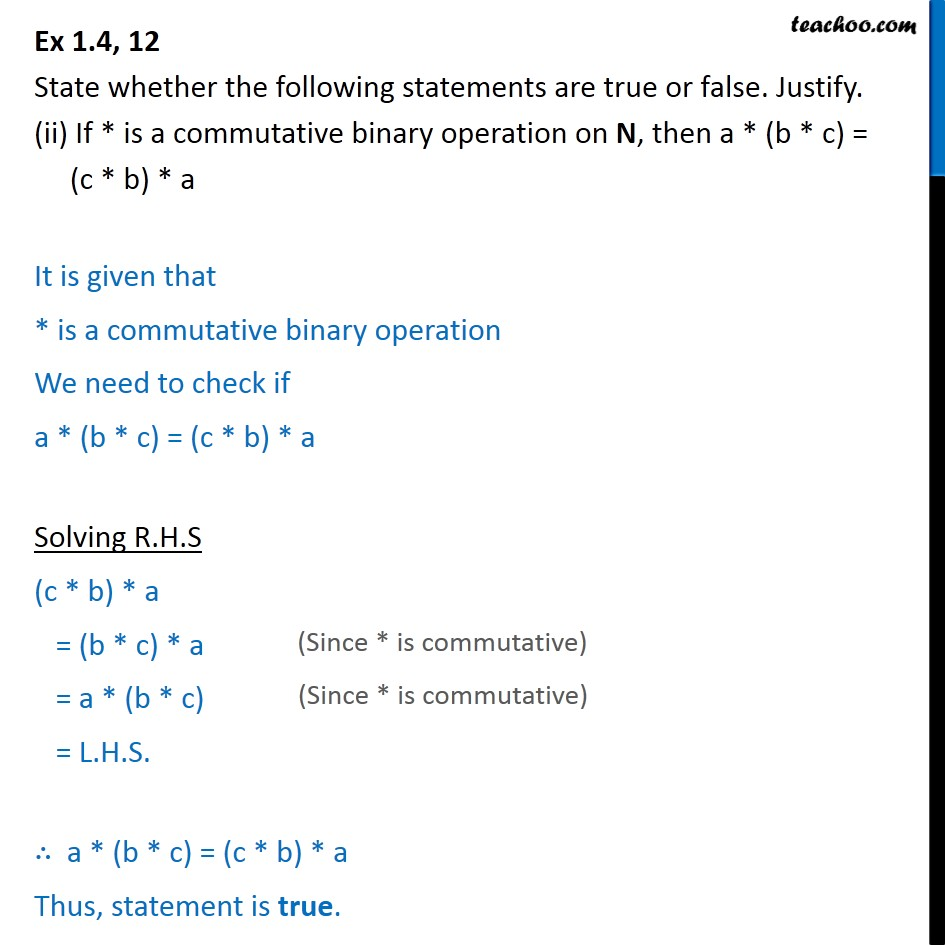Whether binary commutative/associative or not

Chapter 1 Class 12 Relation and Functions
Concept wiseLearn in your speed, with individual attention - Teachoo Maths 1-on-1 Class

### Transcript

Ex 1.4, 12 State whether the following statements are true or false. Justify. (i) For an arbitrary binary operation * on a set N, a * a = a a * N. Let us define * a * b = a + b Calculating a * a Let a = b = 2 So, a * a = 2 * 2 = 2 + 2 = 4 2 So, a * a a Therefore, statement is false. Ex 1.4, 12 State whether the following statements are true or false. Justify. (ii) If * is a commutative binary operation on N, then a * (b * c) = (c * b) * a It is given that * is a commutative binary operation We need to check if a * (b * c) = (c * b) * a Solving R.H.S (c * b) * a = (b * c) * a = a * (b * c) = L.H.S. a * (b * c) = (c * b) * a Thus, statement is true.